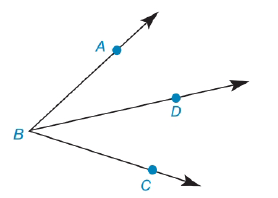Chapter 1.5, Problem 30E### Elementary Geometry for College St...

6th Edition
Daniel C. Alexander + 1 other
ISBN: 9781285195698

#### Solutions

Chapter
Section### Elementary Geometry for College St...

6th Edition
Daniel C. Alexander + 1 other
ISBN: 9781285195698
Textbook Problem
1 views

# In Exercises 27 to 30, fill in the missing reasons for each geometric proof. Given: ∠ A B C and B D → Prove: m ∠ A B D = m ∠ A B C − m ∠ D B C PROOF Statements Reasons 1. ∠ A B C and B D → 1. ? 2. m ∠ A B D + m ∠ D B C = m ∠ A B C 2. ? 3. m ∠ A B D = m ∠ A B C − m ∠ D B C 3. ?Exercises 29, 30

To determine

To find:

The missing reasons for the geometric proof.

Explanation

Given:

The given table is shown below.

 PROOF Statements Reasons 1. ∠ABC and BD→ 1. ? 2. m∠ABD+m∠DBC=m∠ABC 2. ? 3. m∠ABD=m∠ABC−m∠DBC 3. ?

Properties:

1. According to Angle-Addition postulate, if point B lies in the interior of AOC then, mAOB+mBOC=mAOC.

2. The Subtraction Property of Equality states that if a number is subtract from one side of an equation then the same number must be subtracted from the other side of equation to maintain the equality of the original equation.

Calculation:

The first statement of the proof is given below.

ABC and BD

Therefore, the first reason of the proof is Given.

Now, go from first statement to the second statement.

ABC and BD

According to Angle-Addition Postulate, the sum of the measures of ABD and DBC is equal to the measure of ABC as D is the interior of ABC.

mABD+mDBC=mABC

Therefore, the second reason is Angle-Addition Postulate

### Still sussing out bartleby?

Check out a sample textbook solution.

See a sample solution

#### The Solution to Your Study Problems

Bartleby provides explanations to thousands of textbook problems written by our experts, many with advanced degrees!

Get Started

#### Find more solutions based on key concepts